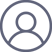姬扬2021-5-13 08:04

1. 中国科学院半导体研究所, 半导体超晶格国家重点实验室, 北京 100083

2. 中国科学院大学, 材料科学与光电技术学院, 北京 100049

Abstract: Here is a square problem: in a unit square, is there a point with four rational distances to the vertices? A probability argument suggests a negative answer. This paper proves several special cases of the square problem: if the point sits on the diagonal , the middle or the side lines of the square, or the side-length of the square is $n$ times the distance from the point to one side (both $n$ and $n^2 + 4$ are prime numbers), the distances from this point to the four vertices can not be all rational. However, this paper does not prove a more general situation. The proof here can be extended to the whole plane, instead of being limited to the interior of the square.

Key words: discrete geometry; rational distance; a square problem

email: jiyang@semi.ac.cn

ChinaXiv版本：

arXiv版本：

Several special cases of a square problem 2105.05250.pdf

[Submitted on 12 May 2021]

Several special cases of a square problem

Yang Ji

Note added in the revised version. I thank Michel Marcus for telling me the following information.

1. Theorem 1  (for the boundary points) is not new. See, 95.01 The rational distance problem, ROY BARBARA, The Mathematical Gazette, Vol. 95, No. 532 (March 2011), pp. 59-61 (3 pages)

Published By: The Mathematical Association. https://www.jstor.org/stable/23248619.

2. For the parameter formula mentioned in Remark 2, see A215365 of the OEIS (https://oeis.org/A215365).

3. Theorem 3 needs both $p$ and $p^2+4$ to be prime number. For such prime pairs, see  A062324 and A045637 of the OEIS. The number of such prime pairs is infinite under the Bunyakovsky conjecture. - Charles R Greathouse IV, Jul 04 2011.1. 正方形问题：在单位正方形的内部，是否存在一个点 P，使得 P 到四个顶点的距离都是有理数？即，线段 PA、PB、PC 和 PD 的长度都是有理数。

$$\begin{array}{c} \left(e-a^{2}-b^{2}\right)=2 a_{1}^{2} b_{1}^{2} \\ \left(e+a^{2}+b^{2}\right)=2^{2 k-1} a_{2}^{2} b_{2}^{2} \end{array}$$

$$e=a_{1}^{2} b_{1}^{2}+2^{2 k-2} a_{2}^{2} b_{2}^{2}$$

$$\begin{array}{r} \left(\left(2^{k} a_{1} a_{2}\right)^{2}+\left(b_{1} b_{2}\right)^{2}\right)^{2}+\left(2^{k} a_{1} a_{2} b_{1} b_{2}\right)^{2} \\ =\left(\left(a_{1} b_{1}\right)^{2}+\left(2^{k-1} a_{2} b_{2}\right)^{2}\right)^{2} \end{array}$$

$$\begin{array}{c} \left(\left(a_{1} b_{1}\right)^{2}-\left(2^{k-1} a_{2} b_{2}\right)^{2}\right)^{2}+\left(2^{k} a_{1} a_{2} b_{1} b_{2}\right)^{2} \\ =\left(\left(a_{1} b_{1}\right)^{2}+\left(2^{k-1} a_{2} b_{2}\right)^{2}\right)^{2} \end{array}$$

$$\left(2^{k} a_{1} a_{2}\right)^{2}+\left(b_{1} b_{2}\right)^{2}=\left(a_{1} b_{1}\right)^{2}-\left(2^{k-1} a_{2} b_{2}\right)^{2}$$

$$\left(a^{2}+b^{2}\right)^{2}+(n a b)^{2}=e^{2}$$

$$\left(\left(a_{1} a_{2}\right)^{2}+\left(b_{1} b_{2}\right)^{2}\right)^{2}+\left(n a_{1} a_{2} b_{1} b_{2}\right)^{2}=e^{2}$$

$$\frac{b^{2}}{a^{2}}=\frac{4 c^{2}+n^{2} d^{2}}{c^{2}-d^{2}}$$

$$\begin{array}{l} \left(n^{2}+4\right) c^{2}=k\left(n^{2} a^{2}+b^{2}\right) \\ \left(n^{2}+4\right) d^{2}=k\left(b^{2}-4 a^{2}\right) \end{array}$$

$$\begin{array}{l} n^{2} a^{2}+b^{2}=c^{2} \\ b^{2}-4 a^{2}=d^{2} \end{array}$$

$$\left(s^{2}+t^{2}\right)^{2}+(n s t)^{2}=c^{2}$$

$$\left(a^{2}+b^{2}\right)^{2}+(n a b)^{2}=e^{2}$$

 C.W. Dodge, Math. Mag. 49, 43 (1976) .

 Math. Mag. 59, 52 (1986).

 Peter Brass, William Moser, Janos Pach, Research Problems in Discrete Geometry, Springer, 2005.

 G. Shute, K.L. Yocom, Math. Mag. 50 , 166 (1977).

 C.W. Dodge, Math. Mag. 49, 43 (1976) . problem 966(iii)

 Math. Mag. 59, 52 (1986). Comments 966 (proposed January 1976; partial solution May 1977). No solution was published for part (iii), which was to determine if it is possible to find a square and an interior point such that the distances from the interior point to the vertices and to the sides are all integers. John P. Robertson (Berkeley, California) has proved that there is no such square if it is required that two of the distances from the point to the sides be equal.

 Peter Brass, William Moser, Janos Pach, Research Problems in Discrete Geometry, Springer, 2005. （第 5.11 节， Integral or Rational DistancesProblem 4, Does there exist a point in the unit square at rational distances from all four vertices? 249 页）. Problem 4 Does there exist a point in the unit square at rational distances from all four vertices? See [DoSY77] for some comments and for the analogous question for arbitrary rectangles. [DoSY77] C.W. Dodge, G. Shute, K.L. Yocom: Seven integral distances. Solution to Problem 966, Math. Mag. 50 (1977) 166 167. Problem 966 posed vol. 49 (1976) p. 43, one further comment appeared in vol. 59 (1986) p. 52.

 C.W. Dodge, G. Shute, K.L. Yocom: Seven integral distances. Solution to Problem 966, Math. Mag. 50, 166-167 (1977). Problem 966 posed vol. 49 (1976) p. 43, one further comment appeared in vol. 59 (1986) p. 52. We have found parametric equations for x, y and a which produce an infinite family of solutions where at least seven of the distances are integral, but we have not found an example where all eight distances are integral.

You say youve got a real solution

Well, you know

Wed all love to see the plan

You ask me for a contribution

Well, you know

We are doing what we can